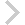FICO Xpress Optimization Examples Repository
 FICO Optimization Community FICO Xpress Optimization HomeRe-solving problem using the Barrier method's warm start

Description
Solve one problem and use its solution to warm-start the solve of a second, slightly different, problem.

Further explanation of this example: 'Xpress Python Reference Manual'

Source Files

example_barrier_warmstart.py

```# Example of the barstart functionality.
#
# (C) Fair Isaac Corp., 2020-2020

import xpress as xp

# Read and solve the first problem

ifdisk = True

p = xp.problem()

p.controls.baralg = 2
p.controls.barstart = 1024
p.controls.crossover = 0

p.lpoptimize('b')

print ('First problem solved with status:', p.getProbStatusString())

if ifdisk:
p.writeslxsol('mysol.slx', 'd')
else:
x, s, d, r = [], [], [], []
p.getlpsol(x, s, d, r)

# Read a slightly modified problem and solve it using the solution of
# p as a warm start.

p2 = xp.problem()

p2.controls.baralg = 2

# This instructs barrier to use the available solution as warm-start.
p2.controls.barstart = -1

p2.controls.crossover = 0

if ifdisk: